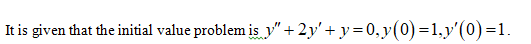# Use the Laplace transform to solve the given initial-value problem.y''+2y'+y=0,    y(0)=1,    y'(0)=1

Question
1 views

Use the Laplace transform to solve the given initial-value problem.

y''+2y'+y=0,    y(0)=1,    y'(0)=1

check_circle

Step 1Step 2

Take Laplace transformation as,

Step 3

On further simpli...

### Want to see the full answer?

See Solution

#### Want to see this answer and more?

Solutions are written by subject experts who are available 24/7. Questions are typically answered within 1 hour.*

See Solution
*Response times may vary by subject and question.
Tagged in

### Math